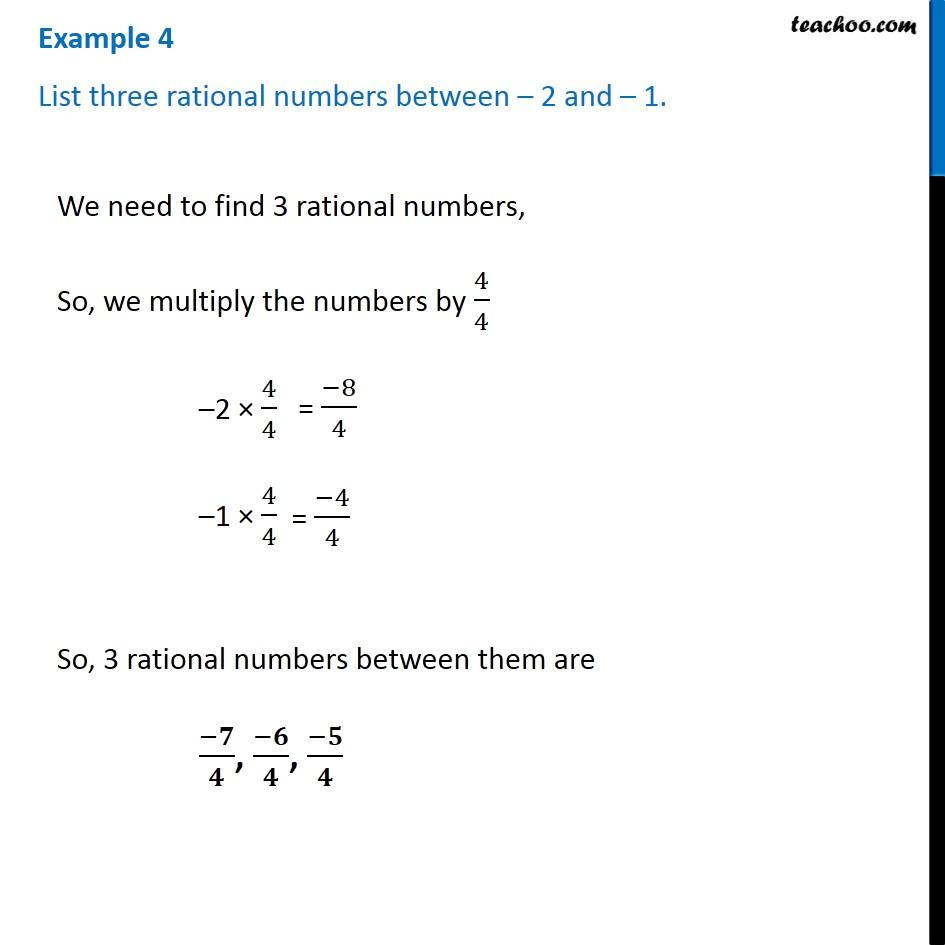Examples

Chapter 8 Class 7 Rational Numbers
Serial order wiseLearn in your speed, with individual attention - Teachoo Maths 1-on-1 Class

### Transcript

Example 4 List three rational numbers between – 2 and – 1. We need to find 3 rational numbers, So, we multiply the numbers by 4/4 –2 × 4/4 –1 × 4/4 So, 3 rational numbers between them are (−𝟕)/𝟒, (−𝟔)/𝟒, (−𝟓)/𝟒